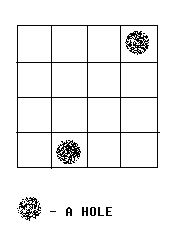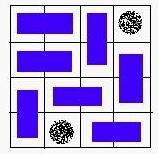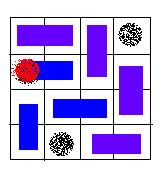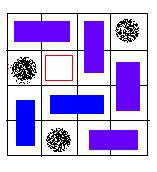Time Limit: 2000MS Memory Limit: 65536KB 64bit IO Format: %lld & %llu

Description

Alice and Bob often play games on chessboard. One day, Alice draws a board with size M * N. She wants Bob to use a lot of cards with size 1 * 2 to cover the board. However, she thinks it too easy to bob, so she makes some holes on the board (as shown in the figure below).We call a grid, which doesn’t contain a hole, a normal grid. Bob has to follow the rules below:
1. Any normal grid should be covered with exactly one card.
2. One card should cover exactly 2 normal adjacent grids.Some examples are given in the figures below:A VALID solution.An invalid solution, because the hole of red color is covered with a card.An invalid solution, because there exists a grid, which is not covered.

Your task is to help Bob to decide whether or not the chessboard can be covered according to the rules above.

Input

There are 3 integers in the first line: m, n, k (0 < m, n <= 32, 0 <= K < m * n), the number of rows, column and holes. In the next k lines, there is a pair of integers (x, y) in each line, which represents a hole in the y-th row, the x-th column.

Output

If the board can be covered, output “YES”. Otherwise, output “NO”.

Sample Input

4 3 2
2 1
3 3


Sample Output

YES

Hint

Source

POJ Monthly,charlescpp

AC代码：
#include <iostream>
#include <cstring>
using namespace std;
const int maxn = 35;
int g[maxn][maxn];
bool hole[maxn][maxn];
int id[maxn][maxn];
int cnt;
bool vis[maxn * maxn];
int m, n, k;
const int dx[] = {0, 0, 1, -1},
dy[] = {1, -1, 0, 0};
void input()
{
cin >> m >> n >> k;
int x, y;
for (int i = 0; i < k; i++)
{
cin >> y >> x;
hole[x][y] = 1;
}
}
bool dfs(int u)
{
for (int v = 1; v <= cnt; v++)
if (g[u][v] && !vis[v])
{
vis[v] = 1;
{
return 1;
}
}
return 0;
}
int hungary()
{
int res = 0;
for (int u = 1; u <= cnt; u++)
{
memset(vis, 0, sizeof(vis));
if (dfs(u))
res++;
}
return res;
}
void solve()
{
for (int i = 1; i <= m; i++)
for (int j = 1; j <= n; j++)
if (!hole[i][j])
{
id[i][j] = ++cnt;
for (int d = 0; d < 4; d++)
if (id[i + dx[d]][j + dy[d]])
g[id[i][j]][id[i + dx[d]][j + dy[d]]] = 1;
}
if (hungary() == cnt)
cout << "YES\n";
else
cout << "NO\n";
}
int main()
{
input();
solve();
return 0;
}